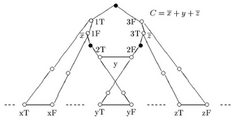## neal young / Hakimi97Orienting

•The paper focuses on two problems: (i) how to orient the edges of an undirected graph in order to maximize the number of ordered vertex pairs $$(x,y)$$ such that there is a directed path from $$x$$ to $$y$$, and (ii) how to orient the edges so as to minimize the number of such pairs. The paper describes a quadratic-time algorithm for the first problem, and a proof that the second problem is NP-hard to approximate within some constant $$1+\epsilon > 1$$. The latter proof also shows that the second problem is equivalent to comparability graph completion''; neither problem was previously known to be NP-hard.